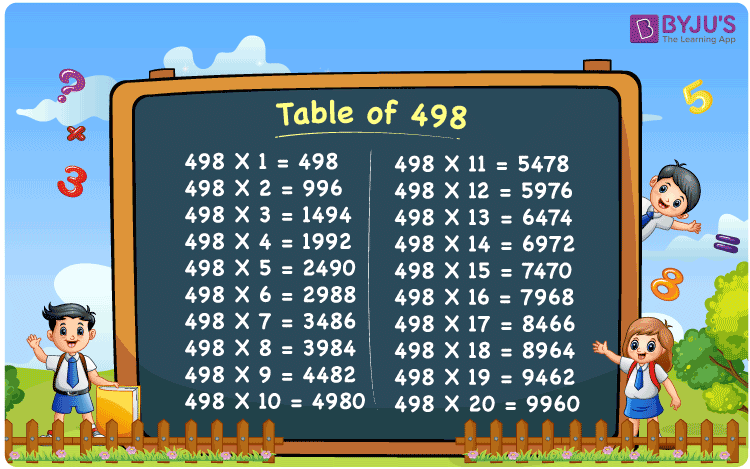Checkout JEE MAINS 2022 Question Paper Analysis : Checkout JEE MAINS 2022 Question Paper Analysis :

# Table of 498

The Table of 498 is provided in a tabular format to help students quickly check the values of multiples of 498. The multiplication table of 498 up to 20 is also available in PDF format to download, which will be handy while solving problems related to the 498 times table. The table of 498 will help while doing mental maths, which is a vital skill for appearing in any competitive examination.

## Table of 498 Chart

The 498 times table chart up to 20 is given here with an attractive image that can be used as a flashcard to memorise the times table of 498. The multiplication table of 498 will help students go through the multiples of 498.## What is the 498 Times Table?

The 498 times table is the multiplication of 498 with any natural number. The 498 times table can be obtained by multiplication and by adding 498 repeatedly. The below chart shows the multiplication table of 498, in both multiplication and addition form.

 498×1 = 498 498 498×2 = 996 498 + 498 = 996 498×3 = 1494 498 + 498 + 498 = 1494 498×4 = 1992 498 + 498 + 498 + 498 = 1992 498×5 = 2490 498 + 498 + 498 + 498 + 498 = 2490 498×6 = 2988 498 + 498 + 498 + 498 + 498 + 498 = 2988 498×7 = 3486 498 + 498 + 498 + 498 + 498 + 498 + 498 = 3486 498×8 = 3984 498 + 498 + 498 + 498 + 498 + 498 + 498 + 498 = 3984 498×9 = 4482 498 + 498 + 498 + 498 + 498 + 498 + 498 + 498 + 498 = 4482 498×10 = 4980 498 + 498 + 498 + 498 + 498 + 498 + 498 + 498 + 498 + 498 = 4980

## Multiplication Table of 498

Given below are the first 20 multiples of 498.

 498 × 1 = 498 498 × 2 = 996 498 × 3 = 1494 498 × 4 = 1992 498 × 5 = 2490 498 × 6 = 2988 498 × 7 = 3486 498 × 8 = 3984 498 × 9 = 4482 498 × 10 = 4980 498 × 11 = 5478 498 × 12 = 5976 498 × 13 = 6474 498 × 14 = 6972 498 × 15 = 7470 498 × 16 = 7968 498 × 17 = 8466 498 × 18 = 8964 498 × 19 = 9462 498 × 20 = 9960

## Solved Example on the Table of 498

Example:

What is two more than the third multiple of 498?

Solution:

The third multiple of 498 = 498×3 = 1494

Two more than 1494 = 1494 + 2 = 1496.

## Frequently Asked Questions on the Table of 498

### What is the times tables of 498?

The 498 times table is the multiplication of 498 with natural numbers. Starting with 498 × 1 = 498, 498 × 2 = 996, 498 × 3 = 1494, 498 × 4 = 1992, 498 × 5 = 2490 and so on.

### What times table has 498?

498 comes in the times tables of 1, 2, 3, 6, 83, 166, 249 and 498.

### What is 5 times 498?

5 times 498 is 2490.